# 7 4 Scientific Notation Goals Use scientific notation

• Slides: 17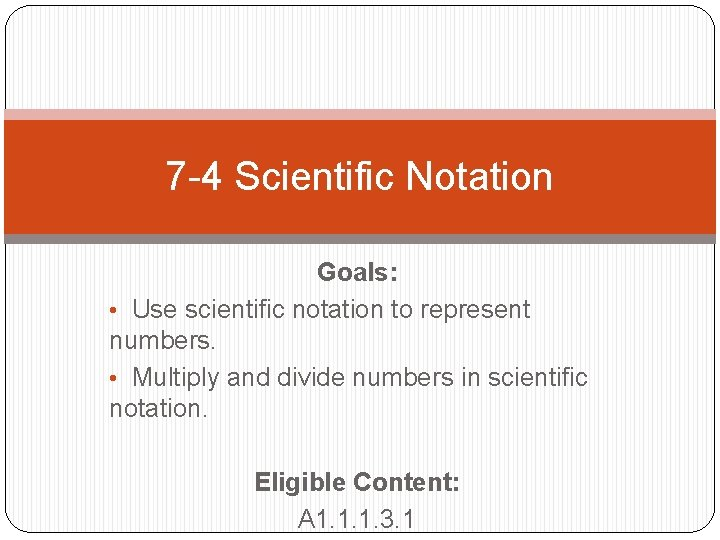7 -4 Scientific Notation Goals: • Use scientific notation to represent numbers. • Multiply and divide numbers in scientific notation. Eligible Content: A 1. 1. 1. 3. 1Vocabulary �Decimal Form – regular numbers. �Examples: � 75, 000 � 0. 0045 � 230 �Scientific Notation – a shorter way to write really big or really small numbers. �Examples: � 6. 02 x 105 � 9. 2 x 10 -5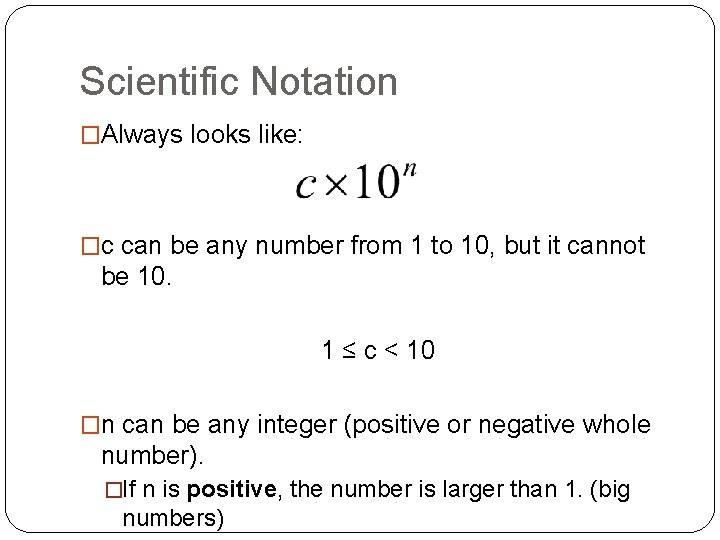Scientific Notation �Always looks like: �c can be any number from 1 to 10, but it cannot be 10. 1 ≤ c < 10 �n can be any integer (positive or negative whole number). �If n is positive, the number is larger than 1. (big numbers)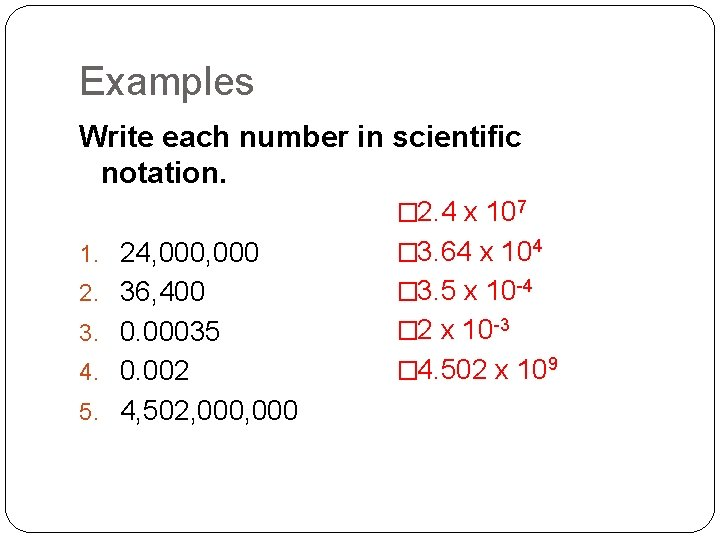Examples Write each number in scientific notation. � 2. 4 x 107 1. 24, 000 � 3. 64 x 104 2. 36, 400 � 3. 5 x 10 -4 3. 0. 00035 � 2 x 10 -3 4. 0. 002 � 4. 502 x 109 5. 4, 502, 000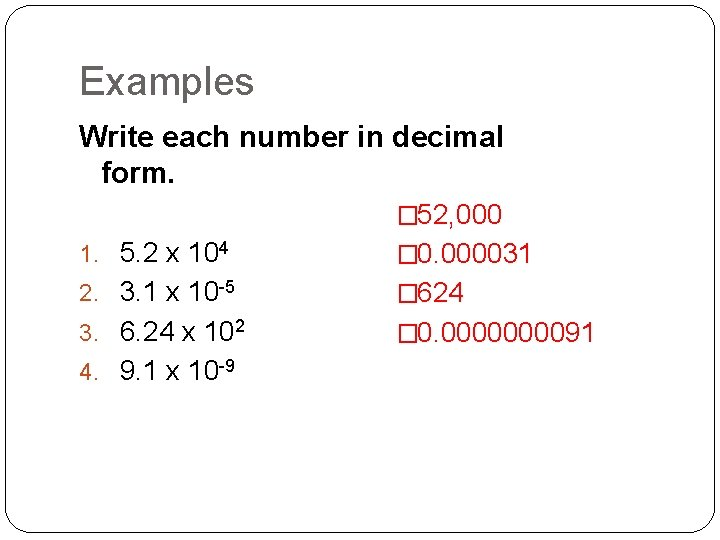Examples Write each number in decimal form. � 52, 000 1. 5. 2 x 104 � 0. 000031 2. 3. 1 x 10 -5 � 624 3. 6. 24 x 102 � 0. 000091 4. 9. 1 x 10 -9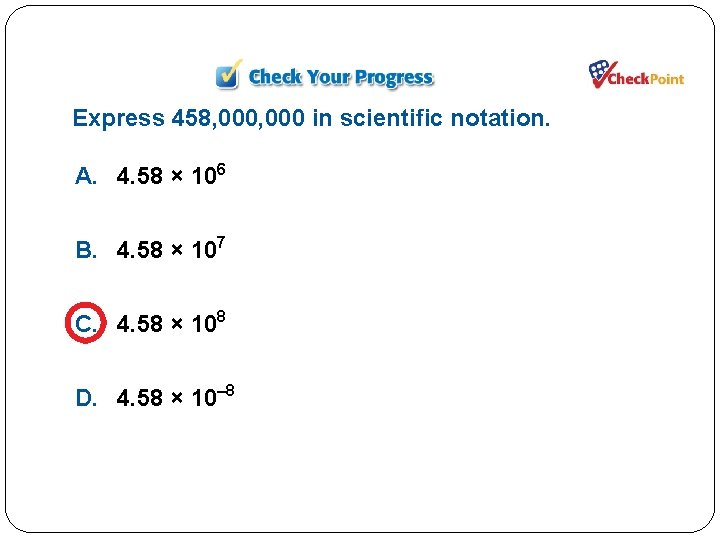Express 458, 000 in scientific notation. A. 4. 58 × 106 B. 4. 58 × 107 C. 4. 58 × 108 D. 4. 58 × 10– 8Express 0. 0000452 in scientific notation. A. 4. 52 × 104 B. 4. 52 × 10– 4 C. 4. 52 × 105 D. 4. 52 × 10– 5Express 3. 16 × 10– 2 in standard notation. A. 0. 0316 B. 0. 00316 C. 3. 1600 D. 316Express 7. 61 × 103 in standard notation. A. 0. 00761 B. 0. 000761 C. 761 D. 7610Practice �Worksheet – “ 7 -4 Scientific Notation” #1 -8Multiplying Numbers in Scientific Notation MULTIPLY numbers ADD exponents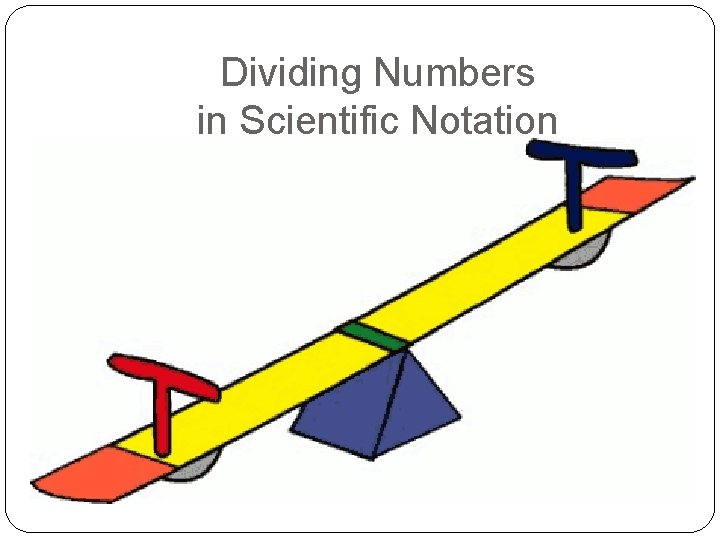Dividing Numbers in Scientific Notation DIVIDE numbers SUBTRACT exponents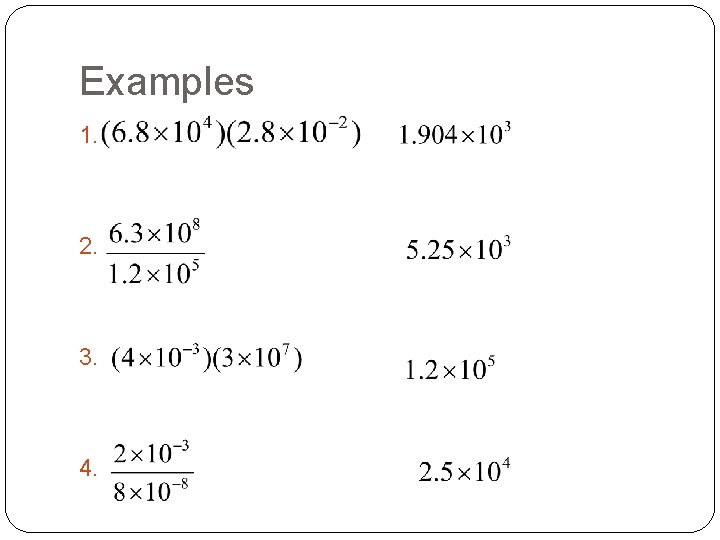Examples 1. 2. 3. 4.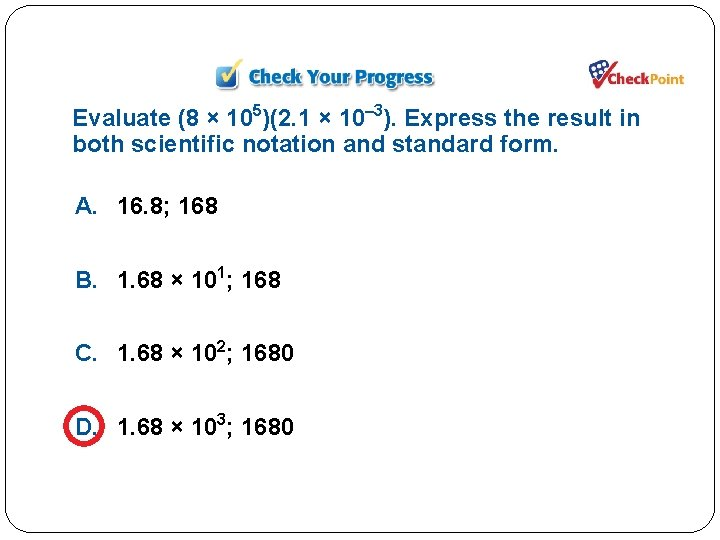Evaluate (8 × 105)(2. 1 × 10– 3). Express the result in both scientific notation and standard form. A. 16. 8; 168 B. 1. 68 × 101; 168 C. 1. 68 × 102; 1680 D. 1. 68 × 103; 1680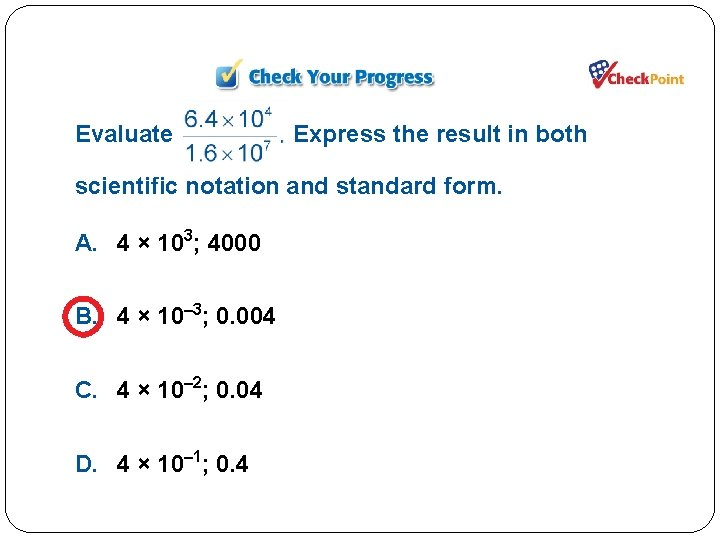Evaluate Express the result in both scientific notation and standard form. A. 4 × 103; 4000 B. 4 × 10– 3; 0. 004 C. 4 × 10– 2; 0. 04 D. 4 × 10– 1; 0. 4Practice Worksheet – “ 7 -2 Scientific Notation” #9 -14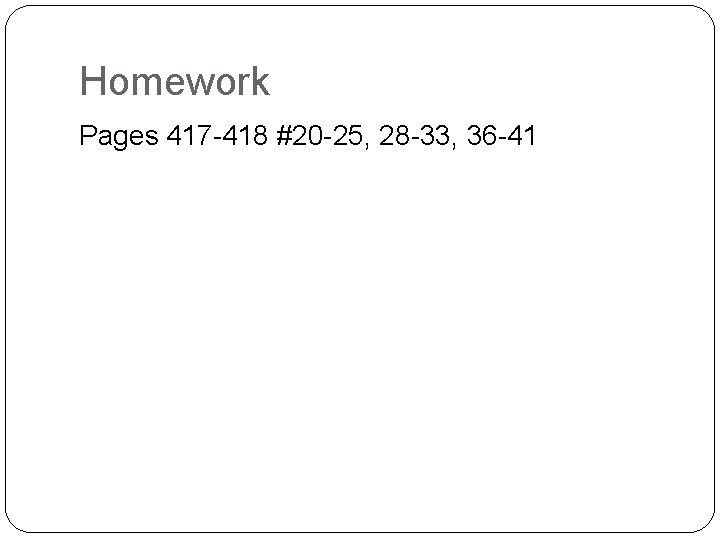Homework Pages 417 -418 #20 -25, 28 -33, 36 -41